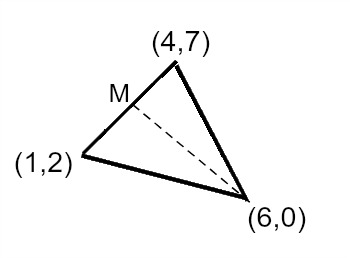# Test Prep Plan - Take a practice test

Take this practice test to check your existing knowledge of the course material. We'll review your answers and create a Test Prep Plan for you based on your results.
How Test Prep Plans work
1
2Based on your results, we'll create a customized Test Prep Plan just for you!
3Study smarter
Study more effectively: skip concepts you already know and focus on what you still need to learn.

# Praxis Middle School Mathematics (5169): Practice & Study Guide Final Exam

Free Practice Test Instructions:

Choose your answer to the question and click 'Continue' to see how you did. Then click 'Next Question' to answer the next question. When you have completed the free practice test, click 'View Results' to see your results. Good luck!

#### Question 2 2. Given the scatterplot shown, which of the following could be the line of best fit of this data?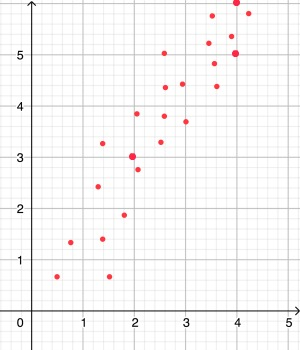#### Question 6 6. The graph of the quadratic function has an equation of the form y = ax^2 + bx + c, where a, b, and c are integers. Find c.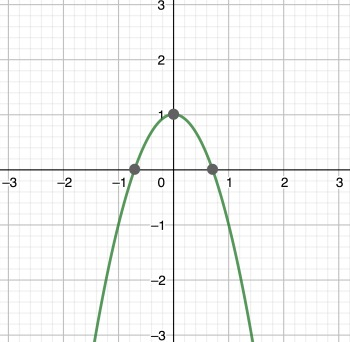#### Question 7 7. The image shows a circular garden. If a farmer wishes to build a fence aroud the outside of the garden, how much fencing will the farmer need?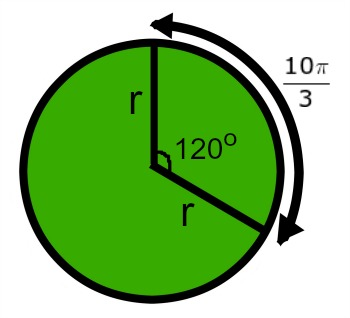#### Question 8 8. The graph of f(x) = x^2 + 2x is shown. What is the domain and range of f(x)?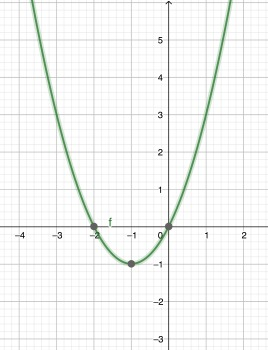#### Question 12 12. The pie chart shows the breakdown of the hair color of the students in a math class. If the number of students with red hair plus the number of students with brown hair is 16, how many students have black hair (round to the nearest whole student).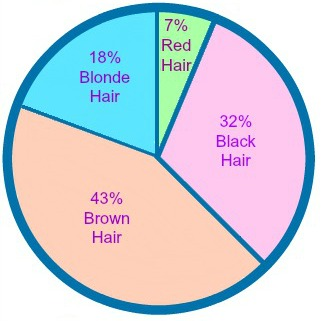#### Question 14 14. What is the slope of the line in the graph?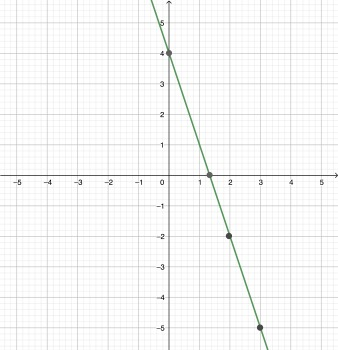#### Question 15 15. The endpoints of a triangle are labeled, and the dotted line is a median of the triangle. Find M with the coordinates in decimal form.